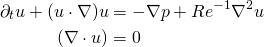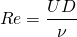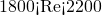# Fluid Dynamics

Fluid dynamics is the study of the motion of fluid. In particular, Computational Fluid Dynamics is the study of this motion using numerical techniques, typically requiring one or more partial differential equations to be solved. One of the most important of these mathematical formulations for describing fluid is the incompressible Navier-Stokes equations## Reynolds number

Many of the substances around us behave as a fluid, even though they may seem to have very different properties such as air, water and syrup. The different behaviours of these substances can be described by a single parameter – the Reynolds number, defined as## Transition

An important consideration in many applications in industry is the transition of a laminar flow to a turbulent state – for instance in flow in a pipe. Driving a turbulent flow in this case requires more energy than driving a laminar flow. Determining the onset of transition, as well as its cause, is therefore a particularly important problem. One useful indicator is given by a linear stability analysis of the flow in which the stability of an infinitesimal disturbance to a steady basic flow is examined under the linearised form of the incompressible Navier-Stokes equations. The evolution of the disturbance can be expressed as a sum of global modes and, if linearly stable, all these global modes decay. However, in practice transition is still observed in some flows which are considered linearly stable.

Contrary to intuition, even if all global modes are decaying, some disturbances may initially grow. This phenomenon is known as transient growth. In some cases this growth may be of many orders of magnitude such as in many of the prototype flows frequently studied. Examples include a sudden expansion in a circular pipe and in flow past a circular cylinder.

### Transition in a Circular Pipe

Transition to turbulence in a straight pipe is found, through experiment and numerical simulation, to take place in the range of Reynolds numbers. In this range, turbulent structures called puffs are found to appear intermittently in an otherwise laminar flow.